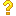•闪存
•博客
• 发言小组
• 投递新闻
• 提问博问
• 添加收藏
• 发布招聘
•文库

0

2

##50初学者，期待大佬给我指点一下

clear t=0:0.01:10; t1=1;%突变点 u1=stepfun(t,t1);%阶跃信号 t2=2; u2=stepfun(t,t2); t3=3; u3=stepfun(t,t3);